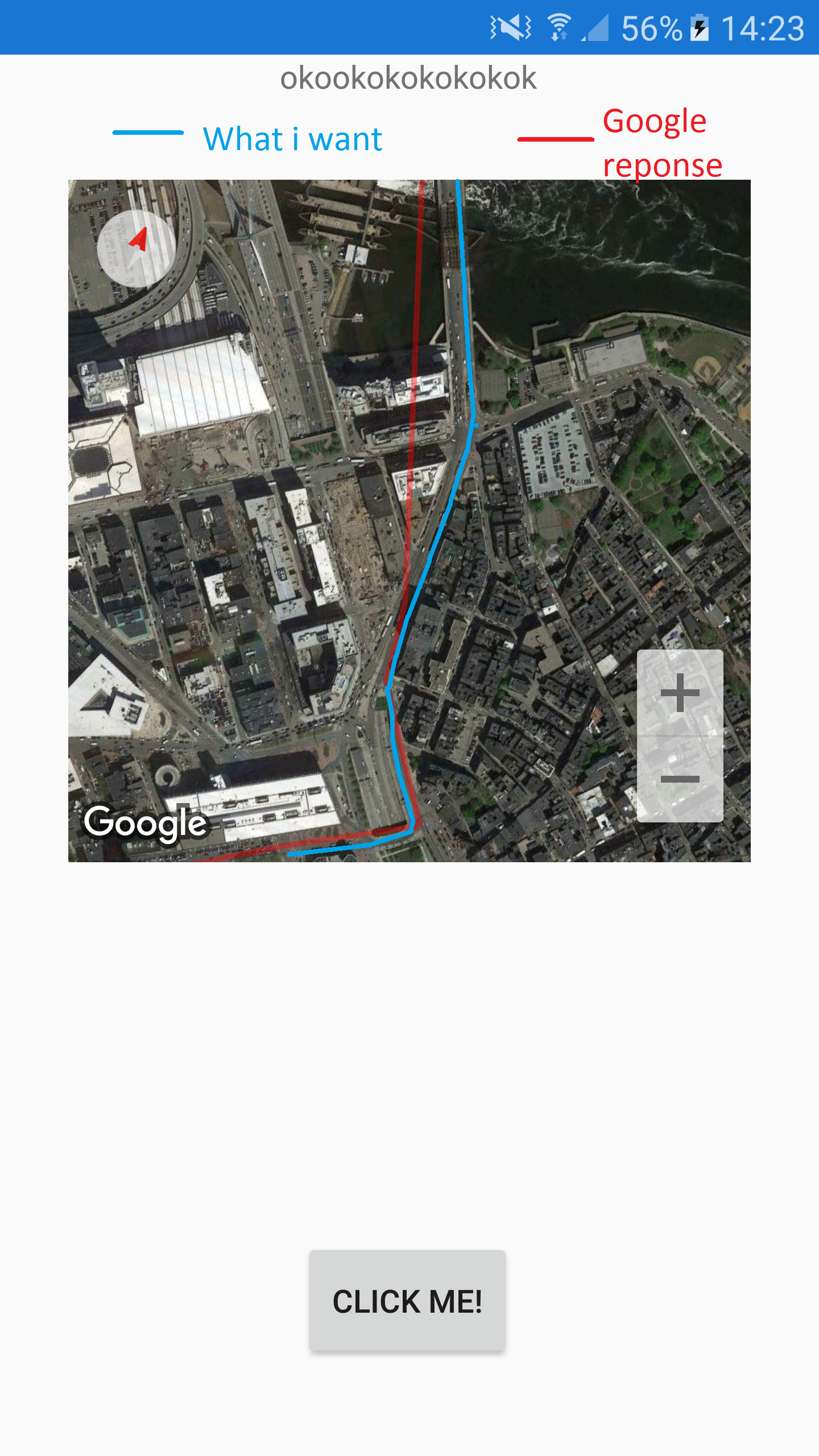# Google Maps - Trace Route Itinerary Direction API

Hi ,

I need trace an itinerary route. I use the Google Direction api for get all point Lat/Lng.. The problème is : # Roads are not a straight line. But the Route is a straightLineHow to do this ? Thx to you !Tagged:

• **I found the solution ! **

The json return the latitude and longitude of all position. for get the curve of the road you can use this =

`"polyline" : { "points" : "k_mfHm_aNMyEA[C}[email protected]?I?I?GB[" },`

Polyline has been Encoded by google and return multiple point(lat,long) after decode.

**DECODE POLYLINE CROSSPLATFORM **

```   >  private List<Position> DecodePolylinePoints(string encodedPoints)
```
```    {
if (encodedPoints == null || encodedPoints == "") return null;
List<Position> poly = new List<Position>();
char[] polylinechars = encodedPoints.ToCharArray();
int index = 0;
int currentLat = 0;
int currentLng = 0;
int next5bits;
int sum;
int shifter;
try
{
while (index < polylinechars.Length)
{
// calculate next latitude
sum = 0;
shifter = 0;
do
{
next5bits = (int)polylinechars[index++] - 63;
sum |= (next5bits & 31) << shifter;
shifter += 5;
} while (next5bits >= 32 && index < polylinechars.Length);
if (index >= polylinechars.Length)
break;
currentLat += (sum & 1) == 1 ? ~(sum >> 1) : (sum >> 1);
//calculate next longitude
sum = 0;
shifter = 0;
do
{
next5bits = (int)polylinechars[index++] - 63;
sum |= (next5bits & 31) << shifter;
shifter += 5;
} while (next5bits >= 32 && index < polylinechars.Length);
if (index >= polylinechars.Length && next5bits >= 32)
break;
currentLng += (sum & 1) == 1 ? ~(sum >> 1) : (sum >> 1);
Position p = new Position(Convert.ToDouble(currentLat) / 100000.0,
Convert.ToDouble(currentLng) / 100000.0);
}
}
catch (Exception ex)
{
//log
}
return poly;
}
```

Enjoy• Hello @LucasPjo
hm hm rather strange me when i try with this command I OBTAIN WELL the correct way

You have perhpas a problem with the decode json ?

My json is good,And i need do this crossplatform, for android and IOSI see your last link many time..

• **I found the solution ! **

The json return the latitude and longitude of all position. for get the curve of the road you can use this =

`"polyline" : { "points" : "k_mfHm_aNMyEA[C}[email protected]?I?I?GB[" },`

Polyline has been Encoded by google and return multiple point(lat,long) after decode.

**DECODE POLYLINE CROSSPLATFORM **

```   >  private List<Position> DecodePolylinePoints(string encodedPoints)
```
```    {
if (encodedPoints == null || encodedPoints == "") return null;
List<Position> poly = new List<Position>();
char[] polylinechars = encodedPoints.ToCharArray();
int index = 0;
int currentLat = 0;
int currentLng = 0;
int next5bits;
int sum;
int shifter;
try
{
while (index < polylinechars.Length)
{
// calculate next latitude
sum = 0;
shifter = 0;
do
{
next5bits = (int)polylinechars[index++] - 63;
sum |= (next5bits & 31) << shifter;
shifter += 5;
} while (next5bits >= 32 && index < polylinechars.Length);
if (index >= polylinechars.Length)
break;
currentLat += (sum & 1) == 1 ? ~(sum >> 1) : (sum >> 1);
//calculate next longitude
sum = 0;
shifter = 0;
do
{
next5bits = (int)polylinechars[index++] - 63;
sum |= (next5bits & 31) << shifter;
shifter += 5;
} while (next5bits >= 32 && index < polylinechars.Length);
if (index >= polylinechars.Length && next5bits >= 32)
break;
currentLng += (sum & 1) == 1 ? ~(sum >> 1) : (sum >> 1);
Position p = new Position(Convert.ToDouble(currentLat) / 100000.0,
Convert.ToDouble(currentLng) / 100000.0);
}
}
catch (Exception ex)
{
//log
}
return poly;
}
```

Enjoy• @LucasPjo Non pas de boulette, tracasses des que je me leve les conneries commencentje debarque dans le mobile et crois moi je galere...

good luck and see you next time• @LucasPjo

• @LucasPjo hi, can you pls help me, how to get JSON from Direction API ?

• USMember, Forum Administrator, Xamarin Team, University Xamurai
edited June 2018

@htran

Look over the Google docs on how to get the json:

A Directions API request takes the following form:

where outputFormat may be either of the following values:

• json (recommended) indicates output in JavaScript Object Notation (JSON)
• xml indicates output as XML

And you can see an example of the REST request in the accepted answer from LucasPjo:

```https://maps.googleapis.com/maps/api/directions/json?origin=Parc%20Naturel%20régional%20du%20Gâtinais%20Français,%20Maison%20du%20Parc%2020%20boulevard%20du%20Maréchal%20Lyautey,%2091490%20Milly-la-Forêt&destination=Chemin%20du%20Rûcher,%2077630%20Arbonne-la-Forêt&mode=driving&key=YOUR_API_KEY_HERE
```

as you can see the outputFormat parameter is json and then they set the origin and destination parameters, a mode parameter, and a key parameter which you will have to get yourself as per the guide linked above.

EDIT: It looks like you started a new discussion on this here: https://forums.xamarin.com/discussion/128240/how-to-link-up-to-google-direction-api#latest
Please try to avoid duplicate posts and since this was bringing up an old post (> 6 months old) starting a new discussion/question is the best option rather than reviving an old thread.